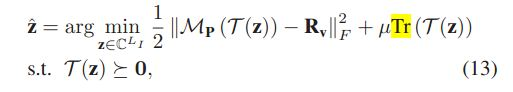# How to resolve norm and trace minimize problem using CVX?

Hi All,
can anybody help me to resolve the following norm and trace minimization probelm using CVX? my matalb code as follow:

cvx_begin
variable ZZ(LI,1) complex
minimize 1/2 * square_pos(norm((P_matrix .* toeplitz(ZZ) - RRV_INTER) , ‘fro’)) +
1/4 * trace(toeplitz(ZZ))
subject to
toeplitz(ZZ) == semidefinite(LI);
cvx_end

error information as following:
error use +
Disciplined convex programming error:
Illegal operation: {convex} + {complex affine}

error minimize
x = evalin(‘caller’,sprintf(’%s’, varargin{:}))

I would appreciate it if anybody can help me to solve this problem.
Thanks
Xie

Given that `Z` is declared to be complex, `1/4 * trace(toeplitz(ZZ))` is complex. Therefore, it can not be an additive term of the objective function. I believe this is what the error message is referring to as `{complex affine} .

I don’t know what problem you actually want to solve, so I can not tell you the correct fix to make. But among other things, the objective function must evaluate to a real scalar. You are allowed to use `real()` in order to accomplish that.

the formula is as follow:I forgot to mention that if ZZ is xomplex, I think you will need to use `toeplitz(ZZ) == hermitian_semidefinite(LI);` instead of `toeplitz(ZZ) == semidefinite(LI); .`

thank you very much for your reply, but how to write the CVX as the formula given above?

It’s your problem, so you will have to figure out the right way of implementing (13). Either don’t declare Z complex, or use real(trace(…)) or whatever else you figure out.

As I wrote above, the objective must evaluate to a real scalar. If you have an optimization problem which doesn’t satisfy that, you will have to figure out what to do.

Thank youvery much, I will have a try